## Schedules in DBMS-

Before you go through this article, make sure that you have gone through the previous article on Transactions in DBMS.

 The order in which the operations of multiple transactions appear for execution is called as a schedule.

## Types of Schedules-

In DBMS, schedules may be classified as-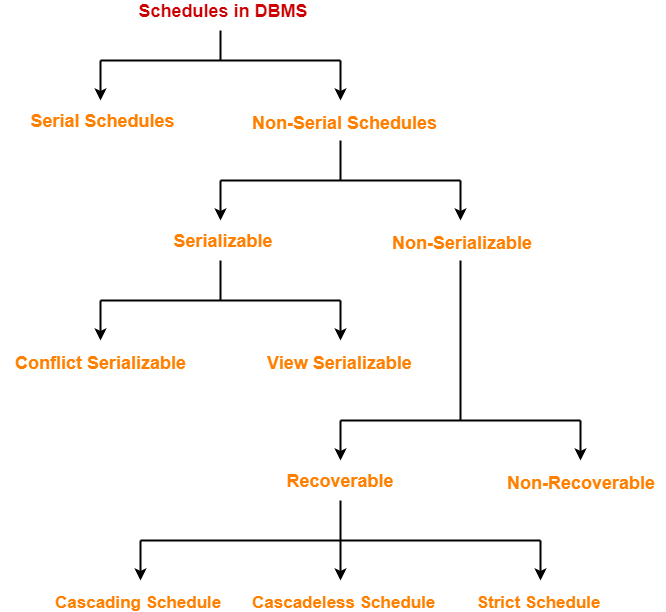## Serial Schedules-

In serial schedules,

• All the transactions execute serially one after the other.
• When one transaction executes, no other transaction is allowed to execute.

## Characteristics-

Serial schedules are always-

• Consistent
• Recoverable
• Strict

### Example-01: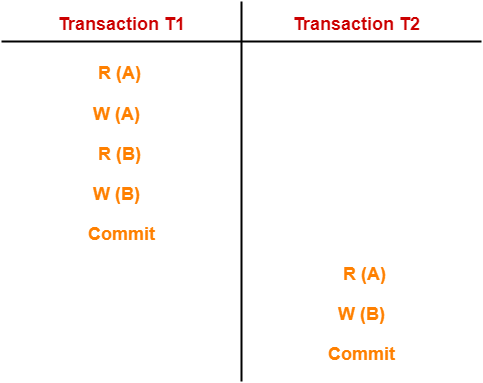In this schedule,

• There are two transactions T1 and T2 executing serially one after the other.
• Transaction T1 executes first.
• After T1 completes its execution, transaction T2 executes.
• So, this schedule is an example of a Serial Schedule.

### Example-02: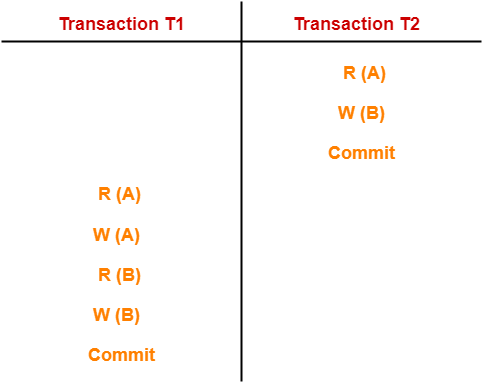In this schedule,

• There are two transactions T1 and T2 executing serially one after the other.
• Transaction T2 executes first.
• After T2 completes its execution, transaction T1 executes.
• So, this schedule is an example of a Serial Schedule.

## Non-Serial Schedules-

In non-serial schedules,

• Multiple transactions execute concurrently.
• Operations of all the transactions are inter leaved or mixed with each other.

## Characteristics-

Non-serial schedules are NOT always-

• Consistent
• Recoverable
• Strict

### Example-01: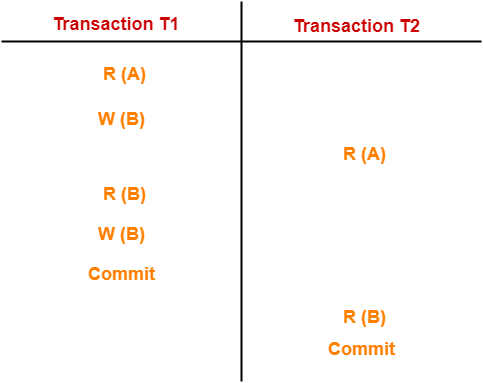In this schedule,

• There are two transactions T1 and T2 executing concurrently.
• The operations of T1 and T2 are interleaved.
• So, this schedule is an example of a Non-Serial Schedule.

### Example-02: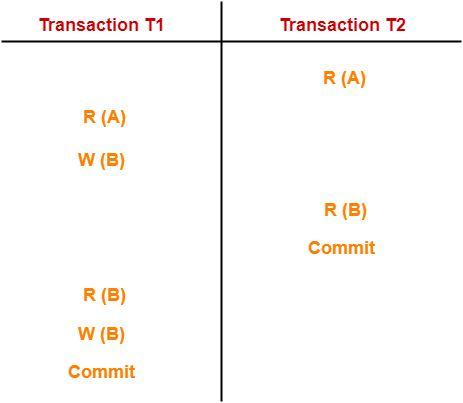In this schedule,

• There are two transactions T1 and T2 executing concurrently.
• The operations of T1 and T2 are interleaved.
• So, this schedule is an example of a Non-Serial Schedule.

## Finding Number Of Schedules-

Consider there are n number of transactions T1, T2, T3 …. , Tn with N1, N2, N3 …. , Nn number of operations respectively.

### Total Number of Schedules-

Total number of possible schedules (serial + non-serial) is given by-### Total Number of Serial Schedules-

Total number of serial schedules

= Number of different ways of arranging n transactions

= n!

### Total Number of Non-Serial Schedules-

Total number of non-serial schedules

= Total number of schedules – Total number of serial schedules

## Problem-

Consider there are three transactions with 2, 3, 4 operations respectively, find-

1. How many total number of schedules are possible?
2. How many total number of serial schedules are possible?
3. How many total number of non-serial schedules are possible?

## Solution-

### Total Number of Schedules-

Using the above formula, we have-### Total Number of Serial Schedules-

Total number of serial schedules

= Number of different ways of arranging 3 transactions

= 3!

= 6

### Total Number of Non-Serial Schedules-

Total number of non-serial schedules

= Total number of schedules – Total number of serial schedules

= 1260 – 6

= 1254

Next Article- Serializability in DBMS

Get more notes and other study material of Database Management System (DBMS).

Watch video lectures by visiting our YouTube channel LearnVidFun.